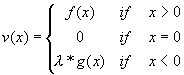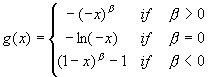Program for Calculating the Cumulative-Prospect-Theory Value
of Prospects with at Most Four Outcomes

The program calculates the value of prospects with no more than four outcomes under cumulative prospect theory (PT), the theory introduced by T&K:

Tversky, Amos & Daniel Kahneman (1992) "Advances in Prospect Theory: Cumulative Representation of Uncertainty," Journal of Risk and Uncertainty 5, 297-323.

You can replace the outcomes, probabilities, and parameters by the ones that you want to consider. The PT value and the certainty equivalent of the prospect under consideration will be given, as well as the decision weights of the outcomes.

Parameters:
 α: (power for gains; 0.88 in T&K). β: (power for losses; 0.88 in T&K). λ: (loss aversion; 2.25 in T&K). γ: (probability weighting parameter for gains; 0.61 in T&K). δ: (probability weighting parameter for losses; 0.69 in T&K).

 First Outcome: with Probability: Second Outcome: with Probability: Third Outcome: with Probability: Fourth Outcome: with Probability:

 PT-Value: Certainty Equivalent:

 Decision Weight of First Outcome: Decicion Weight of Second Outcome: Decision Weight of Third Outcome: Decision Weight of Fourth Outcome:

Elucidation about the Parametric Families:

The weighting function for gains is:                        The weighting function for losses is:The loss aversion parameter is λ

The value function (including loss aversion) is:with functions f(x) and g(x) as follows:written by Veronika Köbberling, November 2002